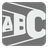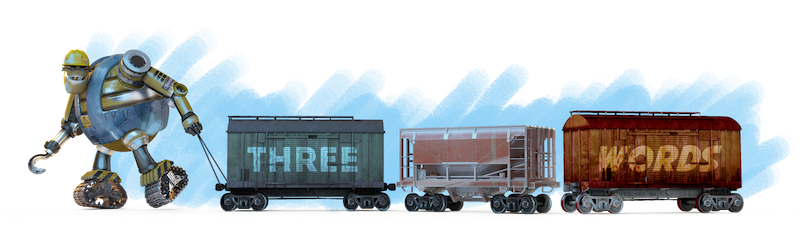Three Words
Elementary
We have prepared a set of Editor's Choice Solutions. You will see them first after you solve the mission. In order to see all other solutions you should change the filter.Let's teach the Robots to distinguish words and numbers.

You are given a string with words and numbers separated by whitespaces (one space). The words contains only letters. You should check if the string contains three words in succession . For example, the string "start 5 one two three 7 end" contains three words in succession.

Input: A string with words.

Output: The answer as a boolean.

Example:

```checkio("Hello World hello") == True
checkio("He is 123 man") == False
checkio("1 2 3 4") == False
checkio("bla bla bla bla") == True
checkio("Hi") == False
```

How it is used: This teaches you how to work with strings and introduces some useful functions.

Precondition: The input contains words and/or numbers. There are no mixed words (letters and digits combined).
0 < len(words) < 100